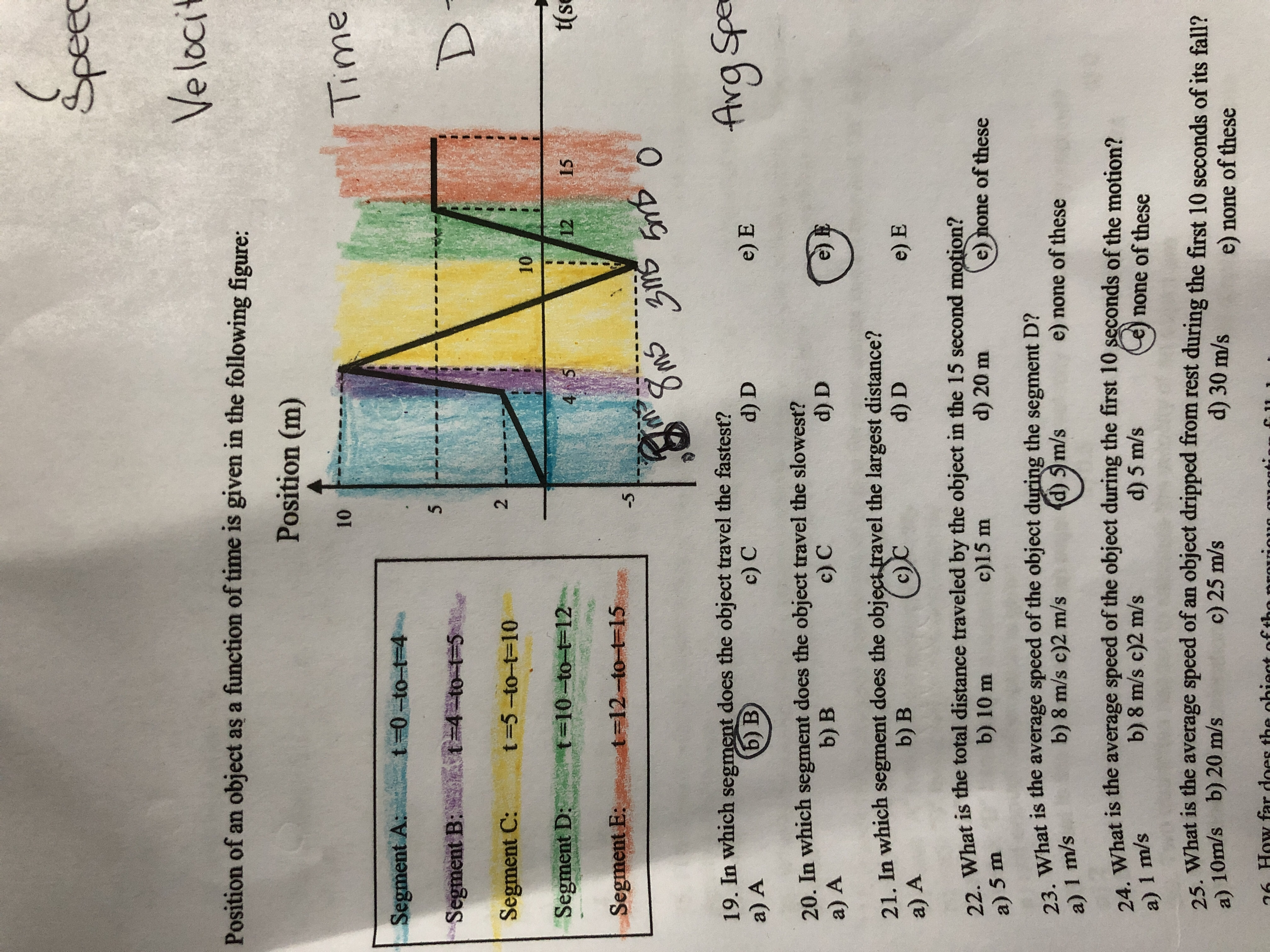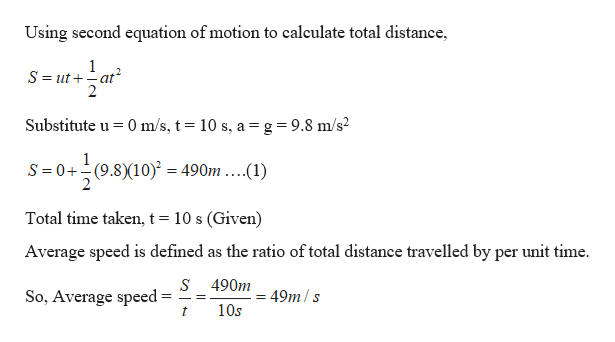# (SpeecVelociPosition of an object as a function of time is given in the following figure:Position (m)10TimeSegment A:t-0-to t 4 at 4 to--5Segment B:DSegment C:t-5-to-t-10210Segment D: t-10-to-t-124 5t(se1215Segment E:t-12 to t 15-5O8ms19. In which segment does the object travel the fastest?a) Afrg Speb) Bc) Cd) De) E20. In which segment does the object travel the slowest?a) Ab) Bc) Cd) D21. In which segment does the object travel the largest distance?(c)Cb) Bd) Da) Ae) E22. What is the total distance traveled by the object in the 15 second motion?c)15 mb) 10 md) 20 me)none of thesea) 5 m23. What is the average speed of the object during the segment D?b) 8 m/s c)2 m/sd) 5 m/se) none of thesea) 1 m/s24. What is the average speed of the object during the first 10 seconds of the motion?d) 5 m/sb) 8 m/s c)2 m/sa) 1 m/snone of these25 What is the average speed of an object dripped from rest during the first 10 seconds of its fall?a) 10m/s b) 20 m/sc) 25 m/sd) 30 m/se) none of these6 How far does the oi t of thenent

Question

What is the average speed of an object dropped from rest during the first 10 seconds of it's fall?help_outlineImage Transcriptionclose( Speec Veloci Position of an object as a function of time is given in the following figure: Position (m) 10 Time Segment A: t-0-to t 4 a t 4 to--5 Segment B: D Segment C: t-5-to-t-10 2 10 Segment D: t-10-to-t-12 4 5 t(se 12 15 Segment E: t-12 to t 15 -5 O 8ms 19. In which segment does the object travel the fastest? a) A frg Spe b) B c) C d) D e) E 20. In which segment does the object travel the slowest? a) A b) B c) C d) D 21. In which segment does the object travel the largest distance? (c)C b) B d) D a) A e) E 22. What is the total distance traveled by the object in the 15 second motion? c)15 m b) 10 m d) 20 m e) none of these a) 5 m 23. What is the average speed of the object during the segment D? b) 8 m/s c)2 m/s d) 5 m/s e) none of these a) 1 m/s 24. What is the average speed of the object during the first 10 seconds of the motion? d) 5 m/s b) 8 m/s c)2 m/s a) 1 m/s none of these 25 What is the average speed of an object dripped from rest during the first 10 seconds of its fall? a) 10m/s b) 20 m/s c) 25 m/s d) 30 m/s e) none of these 6 How far does the oi t of the ne nt fullscreen
check_circleExpert Solution
Step 1

Given:

Initial velocity of the object = 0 m/s

Acceleration of an object falling freely = g = 9.8 m/s2

Step 2

Calculating the average speed of the o...help_outlineImage TranscriptioncloseUsing second equation of motion to calculate total distance 1 S ut at 2 2 Substitute u 0 m/s, t 10 s, a= g = 9.8 m/s2 S 0+(9.810) = 490m ....(1) Total time taken, t = 10 s (Given) Average speed is defined as the ratio of total distance travelled by per unit time. s 490m49m/s So, Average speed= 10s t fullscreen

### Want to see the full answer?

See Solution

#### Want to see this answer and more?

Solutions are written by subject experts who are available 24/7. Questions are typically answered within 1 hour*

See Solution
*Response times may vary by subject and question
Tagged in

### Kinematics